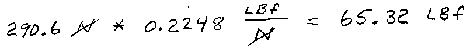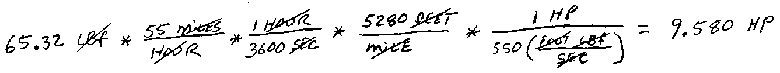# Wind Drag On A Car...

Estimate the drag force, in pounds, on a car with a coefficient of drag of 0.32 and a frontal area of 25 square feet, traveling through air at 55 mph. Then estimate the horsepower required to maintain that speed.

DragForce = 1/2 * Cd * Density * Velocity Squared * Area Power = Force * Velocity

## Without Calchemy

First we must convert the parameters to a coherent system of units so we can actually use the equations. Note the density of air is 1.293 kg/m^3

First convert speed to meters.secThen square feet to square meters...Plug into the equation and get force in Newtons...Then convert Newtons to pounds force...Now calculate the horsepower...## Now the same calculation using CalchemySimple eh?

Calchemy Software Inc.
Fort Collins, COLast Updated 6/21/2009